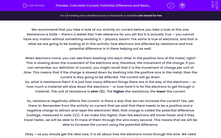# Calculate Current, Potential Difference and Resistance

In this worksheet, students will learn what resistance and voltage are and how they interact.Key stage:  KS 4

Year:  GCSE

GCSE Boards:   AQA, AQA Trilogy, AQA Synergy, OCR 21st Century, OCR Gateway, Pearson Edexcel, Eduqas,

Popular topics:   Physics worksheets, Electricity worksheets

Difficulty level:#### Worksheet Overview

We recommend that you take a look at our activity on current before you take a look at this one.

Resistance is futile – there’s a dated Star Trek reference for you all! But it is actually true – you cannot have any motion without something resisting it – physics, boom! The same is true of electrons, and that is what we are going to be looking at in this activity: how electrons are affected by resistance and how potential difference is in there helping out as well.

When electrons move, you can see them bashing into each other in the positive ions of the metal, right? This is slowing down the movement of the electrons and, therefore, the movement of the charge. If you can remember our definition of current, you might recall that it is the movement of charge per unit of time. This means that if the charge is slowed down by bashing into the positive ions in the metal, then the current is also going to be affected. The current will go down.

So, what is resistance then? It is just how many different things there are in the way of the electrons – or how much a material will slow down the electrons – or how hard it is for the electrons to get through a material. The unit of resistance is ohm (Ω). The higher the resistance, the lower the current.

So, resistance negatively affects the current. Is there a way that we can increase the current? Yes, yes there is! Remember from the activity on current that we said that there needs to be a positive and a negative charge to attract and repel the electrons? Well, that charge is called the potential difference (voltage, measured in volts (V)). If we make this higher, then the electrons will travel faster and if they travel faster, we will be able to fit more of them through the wire every second. This means that we will be able to increase the current using the potential difference.

Okay – so you should get the idea now, it is all about how the electrons move through the wire. We need to be able to predict this movement when we are working with circuits, and so we have come up with an equation to help us do this:

current = potential difference divided by resistance

This can be shown by the formula triangle below: V represents the potential difference  (measured in volts), I represents the current (measured in amps) and R represents the resistance (measured in ohms)The unit of resistance is ohm (Ω), current (ampere, A), voltage (volt, V)

Uses of resistance

A piece of wire has a resistance of 5 Ω and melts if the current through it exceeds 8 A. What is the maximum possible voltage that could cross the wire, without melting it?

To answer this, you need to rearrange the equation. For current, it would become:and for voltage, it would be V = I x R. So, in our question V = 8 x 5 = 40 V

Let's do some questions!

### What is EdPlace?

We're your National Curriculum aligned online education content provider helping each child succeed in English, maths and science from year 1 to GCSE. With an EdPlace account you’ll be able to track and measure progress, helping each child achieve their best. We build confidence and attainment by personalising each child’s learning at a level that suits them.

Get started